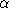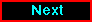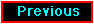# Calibration of Temporal and Spatial Variations of the GIS Gain

## E. Idesawa1, K. Asai2, Y. Ishisaki3, H. Kubo2, A. Kubota1, K. Makishima1, T. Tamura1, M. Tashiro1, and the GIS team

### 1: Department of Physics, University of Tokyo 2: Institute of Space and Astronautical Science 3: Department of Physics, Tokyo Metropolitan University

idesawa@miranda.phys.s.u-tokyo.ac.jp

(This article was unable to be included in the hard copy of the newsletter due to processing problems.)

1 GIS gain correction

The Pulse Height (PH) of each GIS event is corrected by Gain Map for the positional gain dependence, and by temp2gain for the temperature gain dependence and the long-term gain drift (Makishima et al. 1996). In figure 1 we plot the long-term GIS gain drift at the position of 55Fe calibration isotope on the detector rim.

Figure 1 Long-term GIS gain drift at the calibration isotope position. "Cal Peak" indicate the peak pulse height channels of the 55Fe calibration isotope, after correction for the temperature variation. The gain decreased by a few % for the first several months in orbit. At that time the temperature-gain relation coefficients were updated every 10 days, but the trend has gradually flattened. Consequently, we now update the coefficients every 30 days.

So far, we have assumed that the long-term gain trend is similar at any position of the GIS FOV, or equivalently, that the Gain Map is time invariant. However, in recently acquired data, it was noticed that the GIS 3 gain seems to be over-estimated. Accordingly, we have investigated the GIS gain behavior using iron lines from the SNR Cas A, and found that the absolute gain of GIS 3 is apparently overestimated by 1 - 2 % as of August 1995; the deviation is gradually increasing with time, since then.

We have been working to calibrate this newly discovered effect, and found time dependent changes of the Gain Map. Here we report this effect and its correction.

2 The changes of the GIS Gain Map

To examine the Gain Map for possible time dependence, we use the instrumental Cu-Kline at 8.048 keV for calibration. The copper line can be detected over the entire detector plane, although we need a sufficiently long time integration. We divide the detector plane into 25 sectors, and applying temp2gain and the usual Gain Map correction to the raw data, we accumulate Day Earth and Night Earth spectra for every year in each of these regions.

In figure 2, we show the obtained center energy of copper line in 1993--95 for GIS 2 and GIS 3. Thus, the GIS 3 gain gradually becomes overestimated at the detector center as time passes. These effects are thought to originate from gain decreases in the rim region of GIS 3, where the calibration isotope is attached. Because the gain correction has been made referring to the calibration isotope, we have been "overcorrecting" the gain at the center regions of the detector. The gain decrease at the rim region may arise from some decrease in the ultraviolet transmission of the quartz window of the gas cell. These effects are almost negligible in GIS 2.

We find some evidence of azimuthal deviation of the gain from the original gain map. However it is smaller than the radial deviation. Therefore we treat this effect as only depending on the radius, or a distance from the detector center.

Figure 2 Yearly maps summarizing apparent peak energies of the instrumental Cu-Kline originally at 8.048 keV) from the Day/Night Earth spectra, at the 25 separate regions on the GIS detector plane. Each ring has its center at DETX, DETY = (128.5, 128.5), and radii of 0-15, 15-40, 40-70 and 70-10 ch. The origin of (DETX, DETY) is located on the lower left corner. Calibration isotope positions are indicated on maps of 1993 with small circles.

3 GIS Gain Map correction factor

To quantify the relation between radius and gain deviation, we again divide the FOV into one central circle of radius 20 ch, and 8 rings having an equal width of 10 ch covering 20 - 100 ch, and accumulate Day/Night Earth data for every year. The obtained copper line center energy at each ring can be described well with a parabolic function of the radius from the detector center. In figure 3, we show radial distributions of the copper line center energy of GIS 3 in 1993 and 1995.

Then we define a correction factor, a parabolic function of the radius. Dividing by this correction factor, we can correct the pulse invariant (PI) for the time-dependent changes in Gain Map. The specific formula is given as

Correction factor (t) = GMAPC2(t) x r2 + GMAPC0(t)

new PI = oldPI/Correction factor (t)

GMAPC0 indicates the deviation of gain from the original Gain Map at the center of the detector, and GMAPC2, the shape of the parabola. Both GMAPC0 and GMAPC2 are time-dependent coefficients defined separately for GIS 2 and GIS 3. Old PI is Pulse Height corrected for temperature dependence, long term gain drift at the calibration isotope position, and time-constant position dependence (usual Gain Map). New PI is Pulse Height corrected further for the time dependent changes of Gain Map, in addition to those applied to obtain old PI. And r (channel) indicates the distance from the detector center, given as

r2 = (DETX - 128.5)2 + (DETY - 128.5)2

To determine the coefficients GMAPC0 and GMAPC2 for the above parabolic function, we need one year accumulation of Day/Night Earth data for each ring. Therefore, we adopt running average method, and calculate the coefficients for every 6 months. In figure 4 we plot the obtained coefficients GMAPC0 and GMAPC2 as a function of time. These coefficients are written on gis_temp2gain.fits released on 10 Dec 1996 and after.

By interpolating or extrapolating these coefficients given every 6 months, we can get the coefficient at any time. Temp2gain v4.1 released on 18 Dec 1996 reads gis_temp2gain.fits, calculates the Gain Map correction coefficients at the time of observation, and writes them on the header of the gain history file.

Then we can calculate the correction factor at any position. For example, in figure 5 we plot the correction factor in April of 1993 - 1996 as a function of r. Since the temporal gain correction was so far performed referring to the edge of the FOV, where the calibration source is attached, the deviation of the gain is larger in the central region especially in GIS 3. Next version of ASCALIN is planned to read the correction coefficients at the time of observation from the gain history file and perform the correction for each event.

Figure 3 Radial distributions of the instrumental copper line center energy of GIS3, obtained from the Day/Night Earth data. Radius is defined as the distance from the detector center, (DETX, DETY) = (128.5, 128.5). Left panel is for the entire 1993 data, while right panel is for the entire 1995 data.

Figure 4 Time evolution of the coefficients of parabolic function for the time-dependent Gain Map correction, derived from the Day/Night Earth in reference to the copper line energy. GMAPC0 indicates the deviation of the gain at the detector center, and GMAPC2 describes the shape of the parabola.

Figure 5 The correction factor for the time dependence of the Gain Map on four occasions, calculated by interpolating the GMAPC0 and GMAPC2 given in figure 4. The calibration isotope position is indicated with arrows.

Reference

Makishima, K. et al. 1996, PASJ, 48, 171Proceed to the next articleReturn to the previous article